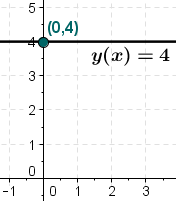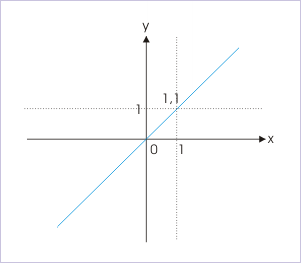Constant and Identity Function for IIT JEE

Definition and Properties of Constant and Identity Function

In this article, we will understand the concept of Constant Function and Identity Function. Let’s start this interesting topic with Constant Function.

Constant Function-

First, we will try to understand this topic with a general example. Any person knows about shopping and has done it. You must have encountered price tag stands with some materials in them. If you haven’t seen such stands, then please consider the below picture as an example. In this picture, everything which is placed under that stand is a set price; moreover you can be lucky enough to find a sale. Now, in this case, a relationship can be established between the items which are under the stand and their prices. This is exactly an example of Constant function.Now it will be easy to understand the mathematical term of constant function. In mathematics, Constant Function is a function in which it doesn’t matter what is the input value because the output value will be the same.

Mathematical Formula of Constant Function- f (x) = a

Here “a” is a number and it is not dependent on x. You all must be wondering how a Constant Function will look like in a graph. Have you ever seen a horizontal line? That is the graphical representation of Constant Function. So in the graphical representation of a Constant Function a horizontal line is included that means a line with slope 0. To elaborate the graphical representation, we can say that in a constant function where y = b , it means that everywhere a Constant Function has a y value and there would be no change in the value of y. So the graph stays constant and would form the horizontal line.
Here one thing should be noted down. It is not mandatory for the single value of a function, like the “a” in the above formula to be a mathematical number like 4, 1, 0 or π. Here what matters it the value of output, which is the same, no matter what value the input variable (x) has.

For example; if the value of y (x) is 4 despite the input value of (x) because function y (x) = 4 is a constant function. To understand this see the below graph:We can consider some other examples like a Constant Function can be written in the form of y = b where b is a constant whose value cannot be changed. That means ‘b’ is a constant. Such as y = 5 or y = 3564 are constant function. Here it doesn’t matter that what x- value or input is, their value or output is always the same.

Simplify Constant Function to understand

The constant function can be understood by simple understanding. Now ask yourself: How can you differentiate between the Constant Function and not a Constant Function? Or can you have different outputs for the different inputs? If you are having different outputs for the different inputs, you don’t have Constant Function. If you are having the same output regardless of the different values of input then only you have Constant Function. We will understand this Non-Constant Function through a example.

Suppose we have y = x+2 or y = x+9. Now, we take the value of x is 2 so output would be y = 2 + 2 or y = 2 + 9
or y = 4 and y = 11
Therefore, the output is varying and input is also different so this is the apt example of a non-constant function.
Suppose we have y = x + 4 or y = x + 3. Now the value of y is 7 no matter what we are putting the value of x (3 or 4). This would be called the perfect example of Constant Function. Here the output is not going to change in spite of having the different input.

Properties of Constant Function-

If someone wants to write a Constant Function formally, it can be written as f (x): R→R and it has the form as f (x) = c. Generally, a Constant Function is written as y (x) = c or it can be written as y = c

• • It has 2 variables x and y in the Constant Function y = c and there is also one constant c in this. Now everyone is wondering why we are including “x” as a variable because it is not written here. But in fact, in this kind of function “x” is always there though we can’t see that.

• • In the Constant Function “c” is a constant which is the real number. We generally replace the “c” with an actual number before working with a linear function.

• • In the third property, it is important to know that any real number “x” can be the input because the input or domain of “y is equal to c is R”. Mathematically we can write it down as y = c is R nonetheless the value “c” is always the output.

• • In the last property, it denotes that “the range of y is always equal to c and c is also R”. The mathematical representation of the above statement is “y = c is which is also R”. Anyhow, the co-domain is just c since the output is always the value of c.

• Some other properties of Constant Function:

• Idempotent is a term which is used when every constant function whose co-domain and domain are the same.
• Second property says that every constant function which is between the topological spaces is continuous.
• The one-point set is a generator in the sets’ category as a corollary.

Identity Function

Everyone knows that functions are widespread and can be seen in mathematics on a daily basis. You must have encountered various kinds of functions in mathematics – such as odd and even functions, surjective function, the identity function, constant function, injective function, bijective function etc. But now we will be focussing on the Identity Function.

First, it is important to know that Identity Function can be called by various names like Identity transformation or Identity relation or Identity map. Identity Function is denoted by “I”. Identity Function is a function in which the same value is given which was earlier used as its input values. Now understand this thing with one simple example: suppose B is a set so the Identity Function “f” on B is defined to be the function with range and domain which assures the relation f (x) = x, for all the elements which are contained in B by x. Now this function f (x) = x is going to be called the “Identity Function” when this function does not change any value of the input of the function. So basically we can say that in Identity Function the same output must be returned by every input value.

The output of the function is the same as the input of the function. The unique function denoted by f-1 is the inverse of the function f and this has the following relationship to f: (f-1 0 f) (x) = x and (f 0 f1) (x) = x

In Identity Function every real number is assigned to this function itself. Here, f (x) = (x) for all x in R defines the function f: R -> R and this is called the Identity Function. R equals to both the range and domain of the Identity Function.

Graphical Representation of Identity Function-

There is no hassle in presenting the graphical representation of the Identity Function. This graphical representation of an identity Function can be defined by f (x) = x for all the values x in R. X – axis takes along the x values and y-axis takes along the f (x) values. The points (x, x) and the points plotted are (x, f(x)) Moreover, by joining all these points we will get a straight line which passes through the origin and is inclined with the x-axis at an angle of 450. After applying this logic, there are certain points on the straight line which are (1, 1), (2, 2), (3, 3)..........etc.Image of Graphical representation of Identity Function

Properties of Identity Function

Properties of any function are the main part of that function so there is a need to describe them in details. Now let’s discuss the properties of Identity Function in brief:

• • The foremost property says Identity Function’s inverse exists which is also an identity Function because Identity Function is injective.

• • The second property says that there is no contradiction between the graph of Identity Function and the graph of Inverse of an identity function. They both will be the same.

• • The third property of identity function is “1 is the slope of the graph of Identity Function”.

• • The fourth property says that the graph of y = x is the same as the graph of an Identity Function.

• • The fifth property says that the graph of Identity Function is consistent over the y = x line.